How would we find a better activation function than ReLU?

Doing to ReLU to what ReLU did to Sigmoid

A neural network is simply a network of connected artificial neurons. We won’t concern ourselves too much with the differences between LSTM, recurrent, convolutional, fully connected, etc — let’s go to the basic unit of the network: the neuron.

The Neuron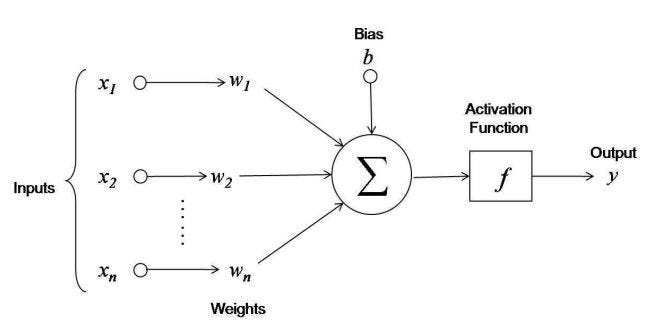Figure 1: A neuron

Looking at Figure 1, we see the basic building block of a neural network. The x’s are the inputs to the neuron. They could be from previous layers or from the actual training or test set of data. For the purposes of a neuron, it doesn’t actually matter — the neuron simply gets 1 or more inputs and multiplies them by the weights, which are the w’s, and then adds those values together. Pretty, simple, right? Then it adds a bias, b, to that sum to arrive at a final value — that’s what that Σ symbol means. But the key to learning is the “activation function,” since that’s what decides whether or not this particular neuron will pass any value along to future neurons (or the final output), and what that value will be.

Activation Function

As stated, the role of the activation function is to convert the value of the summation to something that the rest of the network can use. There are several different activation functions that are used, and are typically denoted by g(z) in the literature. Let’s take a minute to think about the purpose for the activation function before trying to come up with a better one. Let’s first assume that neurons did NOT have activation functions. Then all outputs would be linear and the network as a whole wouldn’t be able to solve anything much greater than a linear equation. Activation functions introduce much needed non linearity so that neural networks can be trained to calculate (potentially any) non linear function.

Sigmoid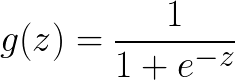Figure 2: Equation for the sigmoid function

One of the most famous activation functions is the sigmoid, which is defined by the equation in Figure 2.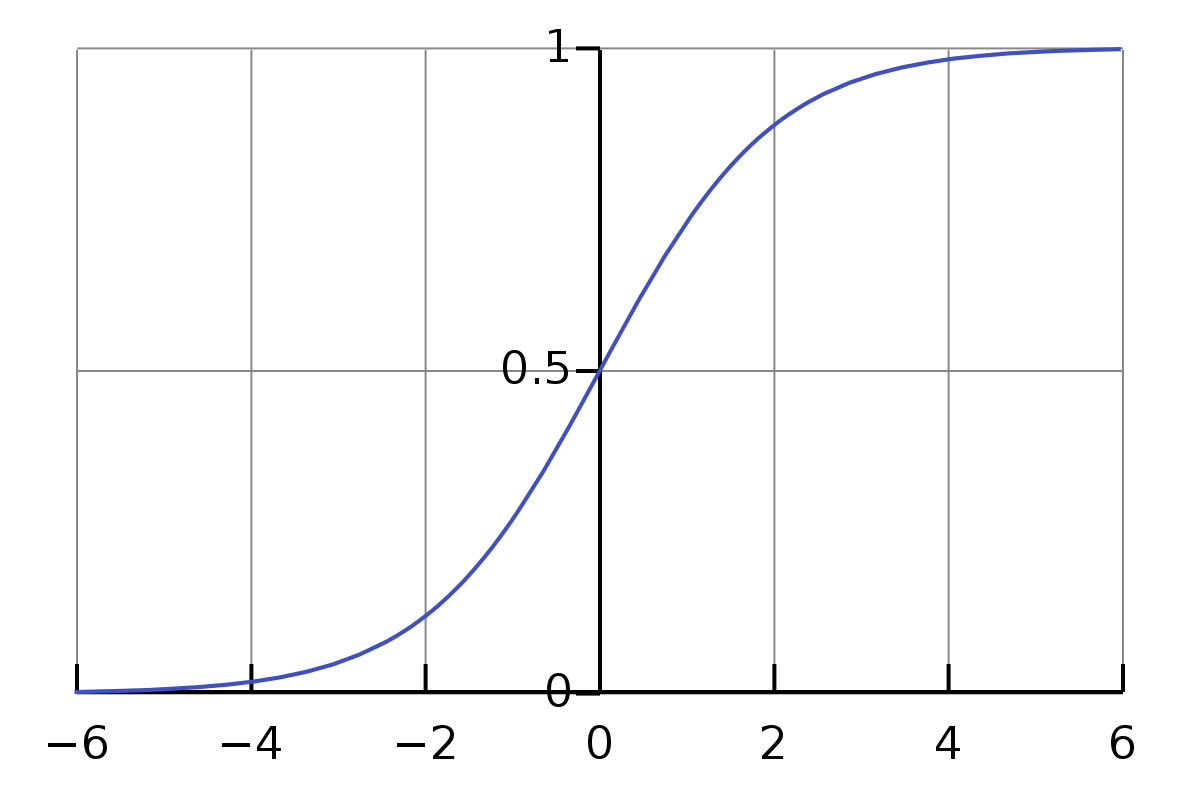Figure 3: Graph of the sigmoid function

When plotted, it looks like the graph shown in Figure 3. It has a maximum that approaches 1 and a minimum that approaches 0. So if we consider the value of our summation from before, no matter how LARGE it gets, once it’s passed to the sigmoid activation function, it gets flattened to a maximum value that approaches 1. This is great for a lot of use cases in which you need to definitely define if something is false (0) or true (1), such as identifying whether an image is of a cat or not.

There are, of course, some problems with the sigmoid function. First, if the value of the summations are extremely large (or small), the change it produces gets smaller and smaller — this is known as a “vanishing gradient.”

tanh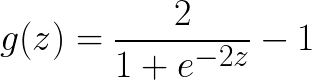Figure 4: tanh activation function

Another closely related, but still different, activation function is the tanh, which is defined by the equation in Figure 4.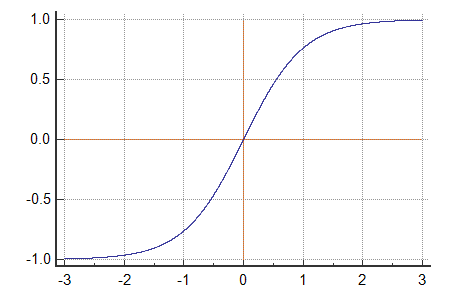Figure 5: tanh graph

Figure 5 shows the graph of the tanh function. You’ll notice that it still has a maximum value of (approaching) 1, but the minimum is now (approaching) -1. This gives the function a steeper gradient, but still suffers from the same “vanishing gradient” problem of the sigmoid. The fact that it allows negative values tends to avoid bias as well, so it is often preferred to the sigmoid.

Rectified Linear Unit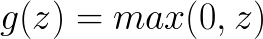Figure 6: ReLU activation function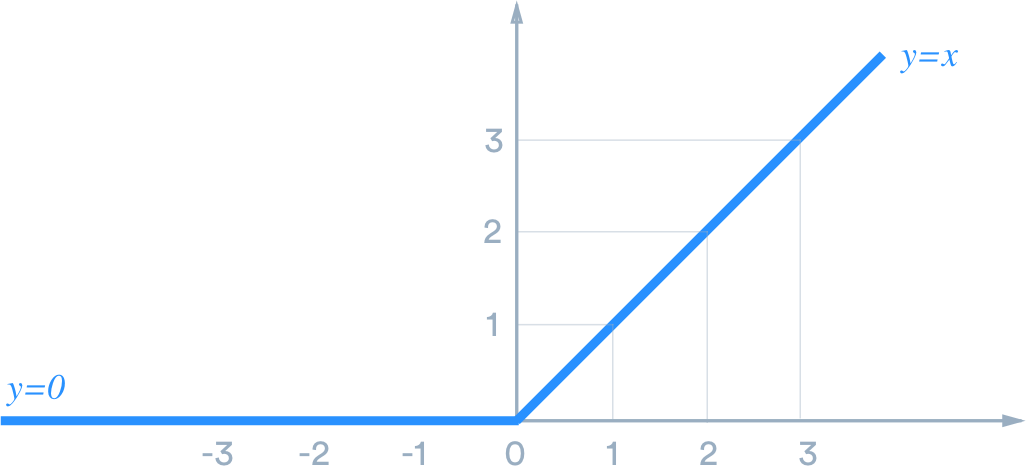Figure 7: Graph of the ReLU function

You’ll notice from the equation in Figure 6 and the graph in Figure 7 that the values are either linear or 0. This has many benefits, including effectively disconnecting neurons that don’t contribute by setting their activation to 0 (don’t propagate a signal). The range of values is also infinite — the activation function will return whatever value is given to it, as long as it’s above 0. Although it was first introduced in the year 2000, but it wasn’t until 2011 that it showed superior performance for deep networks. As of 2018, it is the most popular activation function for deep neural networks.

Handing over the Baton

It seems that almost overnight, other activation functions fell by the way side and ReLU now reigns supreme. This isn’t to say that other activation functions aren’t used, of course — just that there is a clear preference for ReLU given it’s properties and what it enables.

The thing I find most fascinating about ReLU is how simple it is — it eschews complex equations and has proven to be better for training many larger and deeper neural networks.

This is attributed to many things, but chiefly the sparsity and ease of calculation during back propagation. As more and more neurons return a 0, we effectively stop calculating the forward values from that neuron onwards, which leads to an overall sparser network. Since the derivative is constant (a value of 1 in the case above), it solves back propagation algorithms in fewer steps, which leads to the computation cycles being used for more iterations of training rather than derivative calculations.

So is this it — have we found the magical activation formula for deep learning? Neural networks have been around for a looooong time (over 70 years now!) and finally, in the past decade, we have found an activation function that has helped enable miraculous acts of magic that are almost like, but not quite, thinking. Maybe all the researchers out there should just stop? Maybe the top minds working on machine learning problems

Letting the machines take over

I started to wonder about what it would be like for the answer to those questions to be “yes.” Maybe we should stop searching for better activation functions. But that doesn’t mean there won’t be any. What if the machines take over and try to find a better activation function?

Neural Networks to Generate Text

Yup — they exist. It’s possible for machines to generate text that will pass all human tests. With advent of Generative Adversarial Networks, it’s possible to imagine a world in which machines can generate even more compelling text that’s indistinguishable from human generate text.

Machine Generated Equations

It is possible to imagine a world in which machine learning algorithms are able to generate mathematical equations that can be used as activation functions.

They can be of any degree of complexity (or simplicity) and can have any number of bounding conditions. Where as sigmoid activation functions give us a feature of bounding between 0 and 1 and ReLU activation functions give us the benefit of increasing sparsity in the network, a machine generated activation function can give us benefits that we can’t yet imagine.

And testing them would not be overly difficult as there are already well known machine learning problems (such as the MNIST handwriting database) that could be solved with these newly generated activation functions.

Rise of the machinesFigure 8: Terminator

I think we’ll start to see some pretty rapid advancements in artificial intelligence once we take the pesky humans out of the equation. As it is, data scientists around the world spend a ton of time on daily basis tuning hyperparameters, normalizing inputs, evaluating errors and so on. As we start to cede more and more of that authority to machines that are vastly superior in their ability to brute force solutions, I think we’ll see even more rapid progress than this past decade has shown.

Of course, it’s possible that some clever researcher will discover a better activation function, but I think it would be pretty awesome if we let the machines give it a try.